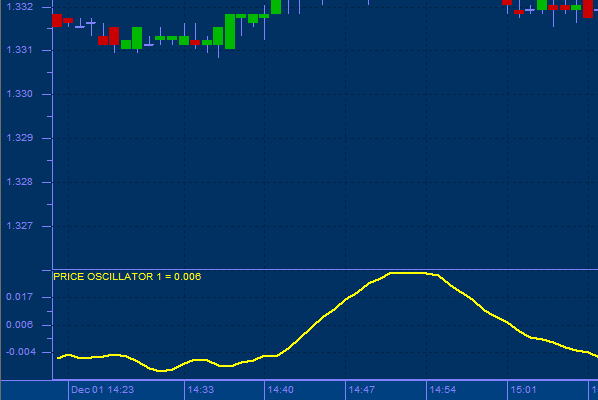## Price Oscillator

The Price Oscillator is an indicator based on the difference between two moving averages and is expressed as either a percentage or in absolute terms. The number of time periods can vary depending on user preference. The Price Oscillator indicator calculates a fast, or short, moving average and a long, or slow, moving average. The difference between these two values is then plotted. The moving averages are not plotted. One approach to analyzing moving averages is to note the relative position of the 2 averages: the short moving average above the long moving average would yield a positive Price Oscillator value and be bullish; the short moving average below the long moving average would yield a negative Price Oscillator value and be bearish.

Usage:

The Price Oscillator illustrates the cyclical and often profitable signals. Moving average analysis typically generates buy signals when a short-term moving average (or the price) rises above a longer-term moving average. Conversely, sell signals are generated when a shorter-term moving average (or the price) falls below a longer-term moving average. Calculating the difference between the two averages and plotting this as an oscillator makes extreme positive and negative values stand out as possible overbought and oversold conditions